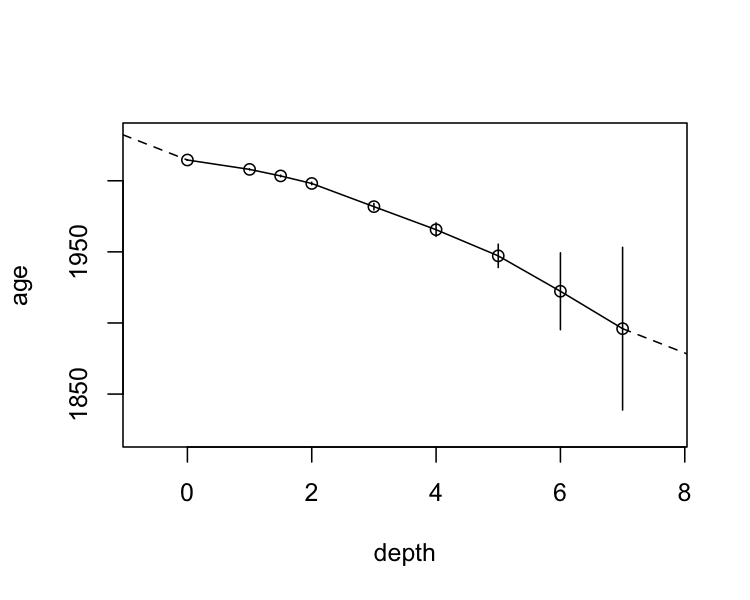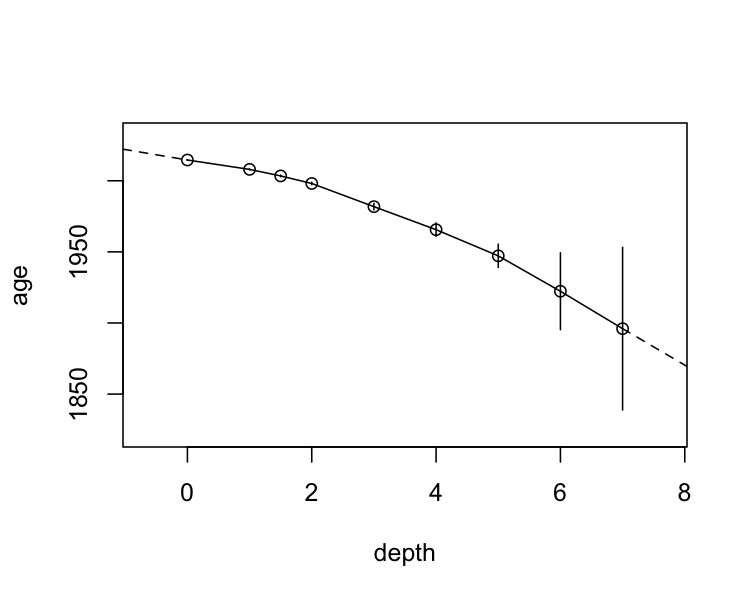# Age-depth models

#### 2022-02-23

Given a known depths and known (or modelled) ages, it is often convenient to approximate age as a continuous function of depth in an archive. This package provides tools to flexibly create age-depth relationships with various rules for interpolating age within known age-depth values, and extrapolating above and below these values. Typically, this is interpolation between known values and extrapolating using average sedimentation rates based on ages known at discrete points in a core.

## Example

Using the built-in dataset alta_lake_210Pb_ages, which contains a Lead-210 (CRS) age-depth relationship for a core from Alta Lake, Whistler, British Columbia, we can create an age-depth model (note that age and depth are evaluated within .data, if it is provided, and support tidy evaluation):

library(tidypaleo)
alta_lake_210Pb_ages,
depth = depth_cm, age = age_year_ad,
)
#> <age_depth_model>
#> Call:
#> age_depth_model(
#>   .data = alta_lake_210Pb_ages, depth = depth_cm,
#>   age_max = age_year_ad + age_error_yr
#> )

Then, we can plot the relationship:

plot(alta_lake_adm)…Or predict raw data:

predict(alta_lake_adm, depth = seq(-1, 10, 0.5))
depth age age_min age_max method
-1.0 2031.701 NA NA extrapolate_above
-0.5 2023.150 NA NA extrapolate_above
0.0 2014.600 2014.60 2014.60 interpolate
0.5 2011.300 NA NA interpolate
1.0 2008.000 2007.66 2008.34 interpolate
1.5 2003.400 2002.84 2003.96 interpolate
2.0 1998.100 1997.24 1998.96 interpolate
2.5 1989.950 NA NA interpolate
3.0 1981.800 1979.55 1984.05 interpolate
3.5 1973.700 NA NA interpolate
4.0 1965.600 1960.87 1970.33 interpolate
4.5 1956.400 NA NA interpolate
5.0 1947.200 1938.99 1955.41 interpolate
5.5 1934.750 NA NA interpolate
6.0 1922.300 1895.28 1949.32 interpolate
6.5 1909.150 NA NA interpolate
7.0 1896.000 1838.81 1953.19 interpolate
7.5 1887.450 NA NA extrapolate_below
8.0 1878.899 NA NA extrapolate_below
8.5 1870.349 NA NA extrapolate_below
9.0 1861.798 NA NA extrapolate_below
9.5 1853.248 NA NA extrapolate_below
10.0 1844.697 NA NA extrapolate_below

The default behaviour is to interpolate within known ages/depths, and extrapolate using a linear fit of ages/depths. These can be specified using transform functions, which take XY data and produce forward and inverse predictions based on them. The default call is:

age_depth_model(
...,
interpolate_age = age_depth_interpolate,
extrapolate_age_below = ~age_depth_extrapolate(.x, .y, x0 = last, y0 = last),
extrapolate_age_above = ~age_depth_extrapolate(.x, .y, x0 = first, y0 = first),
interpolate_age_limits = trans_exact,
extrapolate_age_limits_below = trans_na,
extrapolate_age_limits_above = trans_na
)

To customize the behaviour of the predictions (e.g., disable extrapolating above or below), specify a transform function in the appropriate category. One-sided formulas are turned into functions using the rlang::as_function(). A more advanced way might be to only use the first/last few observations to extrapolate above and below, which one could do like this:

alta_lake_adm2 <- age_depth_model(
alta_lake_210Pb_ages,
depth = depth_cm, age = age_year_ad,
plot(alta_lake_adm2)